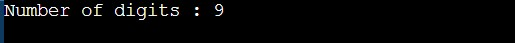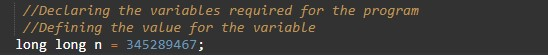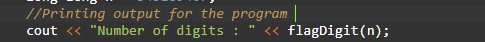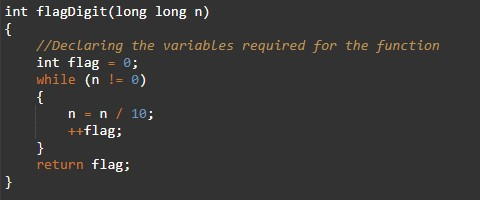# C++ Programs to Find Number Of Digits

In this tutorial you will learn about the C++ Programs to Find Number Of Digits and its application with practical example.

## C++ Program to Find the number of digits.

In this tutorial, we will learn to create a C++ program that will Find the Number Of Digits in C++ programming.

## Prerequisites

Before starting with this tutorial we assume that you are best aware of the following C++ programming topics:

• Operators in C++ Programming.
• Basic Input and Output function in C++ Programming.
• Basic C++ programming.
• String functions in c++ programming.

## Find the number of digits in the number.

As we all know the c++ programming is a very powerful language. In the c++ programming language, we have many pre-defined functions for arithmetic operations. but today we will count the number of digits in a number without using the mod operator.

With the help of this program, we can find the number of digits in numbers.

## Output:-In the above program, we have first initialized the required variable.• n = it will hold the long integer.
• flag = it will hold the integer value for numbers.

Taking input number to the user.Calling function for reverse and printingFinding the number of digits with the help of using a user-defined function.In this tutorial we have learn about the C++ Programs to Find Number Of Digits and its application with practical example. I hope you will like this tutorial.